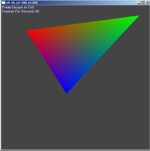Friday September 22, 2023
 home | about | contact | DonationsThe Maths of 3D You can't have 3D without a little maths...

Rasterization : Gouraud Solid Triangle

by bkenwright@xbdev.net

{UnderConstruction}

This is the most popular method of rendering triangles in the world of 3D....99% of all triangle rendering is done using Gouraud shading, so I think we should have a looksy....and believe me it looks cool :)

So how does it work?  Well its very similar to how you would render a sold triangle using interpolation of the coordinates....but as well as interpolating the x and y....we also interpolate the red, green and blue.  Now looking at the code is only going to scare you unless you understand the basics of how to interpolate from one point to the next, so if you've not gone over the previous tutorial on rendering a solid triangle I would recomend doing it first...the code is more or less identical...but this one has loads of colour interpolation stuff added in.

 DownloadSourceCode   Test out the demo program, it should work without any problems - as you move the cursor around your triangle will resize.  Again error checking has been cut down to almost nothing... but it seems okay and has no serious problems.  Hopefully the underlying principles of how it works can be seen in this code.Code: void Draw_Gouraud_Triangle(unsigned int* pBits, int w, int h, int pitch,                         int x0, int y0, float r0, float g0, float b0,                               int x1, int y1, float r1, float g1, float b1,                               int x2, int y2, float r2, float g2, float b2) {       // Sort our points into order of y       // 0 top       // 2 middle       // 1 bottom       if( y1 < y0 )       {             SWAP(y1, y0);             SWAP(x1, x0);             SWAP(r1, r0);  SWAP(g1, g0);  SWAP(b1, b0);       }       if( y2 < y0 )       {             SWAP(y2, y0);             SWAP(x2, x0);             SWAP(r2, r0);  SWAP(g2, g0);  SWAP(b2, b0);       }       if( y1 < y2 )       {             SWAP(y2, y1);             SWAP(x2, x1);             SWAP(r2, r1);  SWAP(g2, g1);  SWAP(b2, b1);       }           float xl_edge = (float)x0;  // left edge       float xr_edge = (float)x0;  // right edge           float dxldy;       float dxrdy;           float dxdy1 = (float)(x2-x0)/(y2-y0);       float dxdy2 = (float)(x1-x0)/(y1-y0);         float dr1 = (float)(r2-r0)/(y2-y0);       float dg1 = (float)(g2-g0)/(y2-y0);       float db1 = (float)(b2-b0)/(y2-y0);         float dr2 = (float)(r1-r0)/(y1-y0);       float dg2 = (float)(g1-g0)/(y1-y0);       float db2 = (float)(b1-b0)/(y1-y0);         float drldy, dgldy, dbldy;       float drrdy, dgrdy, dbrdy;         if( dxdy1 < dxdy2 )       {             dxldy = dxdy1;             dxrdy = dxdy2;               drldy  = dr1;     dgldy  = dg1;     dbldy  = db1; // left  (r,g,b)             drrdy  = dr2;     dgrdy  = dg2;     dbrdy  = db2; // right (r,g,b)         }       else       {             dxldy = dxdy2;             dxrdy = dxdy1;               drldy  = dr2;     dgldy  = dg2;     dbldy  = db2; // left  (r,g,b)             drrdy  = dr1;     dgrdy  = dg1;     dbrdy  = db1; // right (r,g,b)       }         float r_left  = r0;       float r_right = r0;       float g_left  = g0;       float g_right = g0;       float b_left  = b0;       float b_right = b0;         // Top of the triangle       for(int y=y0; y0) && (mousePos.x0) )       {             char buf;             sprintf(buf, "x: %d, y: %d", mousePos.x, mousePos.y);             //SetWindowText(hWnd, buf);               x1 = mousePos.x;             y1 = mousePos.y;       }// End of if mousePos...etc         Draw_Gouraud_Triangle(pBits, w, h, pitch,                         x0, y0, 0xff, 0x00, 0x00, // x,y (point) - red, greed blue (colour)                               x1, y1, 0x00, 0xff, 0x00,                               x2, y2, 0x00, 0x00, 0xff );   }// end of Render(..)

It looks like a lot, and it is... the best thing to do, is to break it up!  Don't let that code intimidate you....as I had to do, I printed it out and spent a while with a pencil doodling some thinking and testing it out on the pc.  But once you understand how it works....the only other thing worth doing is?  is?   what is it?  Well its optimisation.... all of those float to int conversions taking place might need a bit of fixing.... which is what we'll do next.

{ Comming Soon - Using Fixed Point Math For Optimisation }